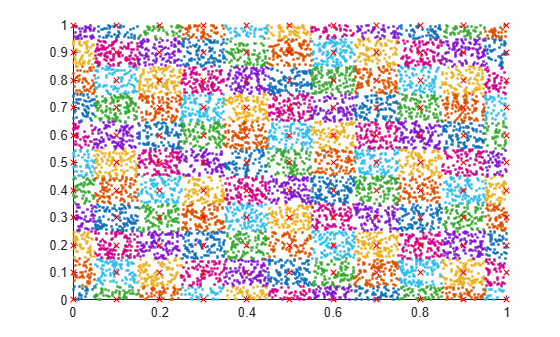# Group Scattered Data Using a Tolerance

This example shows how to group scattered data points based on their proximity to points of interest.

Create a set of random 2-D points. Then create and plot a grid of equally spaced points on top of the random data.

```x = rand(10000,2); [a,b] = meshgrid(0:0.1:1); gridPoints = [a(:), b(:)]; plot(x(:,1), x(:,2), '.') hold on plot(gridPoints(:,1), gridPoints(:,2), 'xr', 'Markersize', 6)```Use `ismembertol` to locate the data points in `x` that are within tolerance of the grid points in `gridPoints`. Use these options with `ismembertol`:

• Specify `ByRows` as `true`, since the point coordinates are in the rows of `x`.

• Specify `OutputAllIndices` as `true` to return all of the indices for rows in `x` that are within tolerance of the corresponding row in `gridPoints`.

```[LIA,LocB] = ismembertol(gridPoints, x, 0.05, ... 'ByRows', true, 'OutputAllIndices', true);```

For each grid point, plot the points in `x` that are within tolerance of that grid point.

```figure hold on for k = 1:length(LocB) plot(x(LocB{k},1), x(LocB{k},2), '.') end plot(gridPoints(:,1), gridPoints(:,2), 'xr', 'Markersize', 6)```